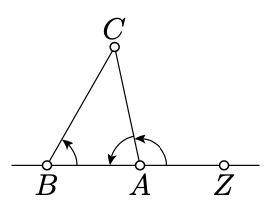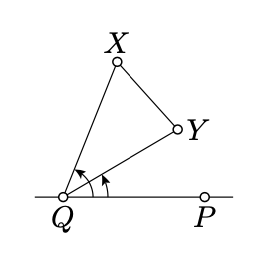$$\newcommand{\id}{\mathrm{id}}$$ $$\newcommand{\Span}{\mathrm{span}}$$ $$\newcommand{\kernel}{\mathrm{null}\,}$$ $$\newcommand{\range}{\mathrm{range}\,}$$ $$\newcommand{\RealPart}{\mathrm{Re}}$$ $$\newcommand{\ImaginaryPart}{\mathrm{Im}}$$ $$\newcommand{\Argument}{\mathrm{Arg}}$$ $$\newcommand{\norm}{\| #1 \|}$$ $$\newcommand{\inner}{\langle #1, #2 \rangle}$$ $$\newcommand{\Span}{\mathrm{span}}$$

# 3.3: Same sign lemmas

$$\newcommand{\vecs}{\overset { \rightharpoonup} {\mathbf{#1}} }$$ $$\newcommand{\vecd}{\overset{-\!-\!\rightharpoonup}{\vphantom{a}\smash {#1}}}$$$$\newcommand{\id}{\mathrm{id}}$$ $$\newcommand{\Span}{\mathrm{span}}$$ $$\newcommand{\kernel}{\mathrm{null}\,}$$ $$\newcommand{\range}{\mathrm{range}\,}$$ $$\newcommand{\RealPart}{\mathrm{Re}}$$ $$\newcommand{\ImaginaryPart}{\mathrm{Im}}$$ $$\newcommand{\Argument}{\mathrm{Arg}}$$ $$\newcommand{\norm}{\| #1 \|}$$ $$\newcommand{\inner}{\langle #1, #2 \rangle}$$ $$\newcommand{\Span}{\mathrm{span}}$$ $$\newcommand{\id}{\mathrm{id}}$$ $$\newcommand{\Span}{\mathrm{span}}$$ $$\newcommand{\kernel}{\mathrm{null}\,}$$ $$\newcommand{\range}{\mathrm{range}\,}$$ $$\newcommand{\RealPart}{\mathrm{Re}}$$ $$\newcommand{\ImaginaryPart}{\mathrm{Im}}$$ $$\newcommand{\Argument}{\mathrm{Arg}}$$ $$\newcommand{\norm}{\| #1 \|}$$ $$\newcommand{\inner}{\langle #1, #2 \rangle}$$ $$\newcommand{\Span}{\mathrm{span}}$$

Lemma $$\PageIndex{1}$$

Assume $$Q' \in [PQ)$$ and $$Q' \ne P$$. Then for any $$X \in (PQ)$$ the angles $$PQX$$ and $$PQ'X$$ have the same sign.Proof

By Proposition 2.2.2, for any $$t \in [0, 1]$$ there is a unique point $$Q_t \in [PQ)$$ such that

$PQ_t = (1 - t) \cdot PQ + t \cdot PQ'.$

Note that the map $$t \mapsto Q_t$$ is continuous,

$$Q_0 = Q$$,                    $$Q_1 = Q'$$

and for any $$t \in [0, 1]$$, we have that $$P \ne Q_t$$.

Applying Corollary 3.2.1, for $$P_t = P$$, $$Q_t$$, and $$X_t = X$$, we get that $$\angle PQX$$ has the same sign as $$\angle PQ'X$$.

Theorem $$\PageIndex{1}$$ Signs of angles of a triangle

In arbitrary nondegenerate triangle $$ABC$$, the angles $$ABC, BCA,$$ and $$CAB$$ have the same sign.Proof

Choose a point $$Z \in (AB)$$ so that $$A$$ lies between $$B$$ and $$Z$$.

According to Lemma $$\PageIndex{1}$$, the angles $$ZBC$$ and $$ZAC$$ have the same sign.

Note that $$\measuredangle ABC = \measuredangle ZBC$$ and

$\measuredangle ZAC + \measuredangle CAB \equiv \pi.$

Therefore, $$\angle CAB$$ has the same sign as $$\angle ZAC$$ which in turn has the same sign as $$\measuredangle ABC = \measuredangle ZBC$$.

Repeating the same argument for $$\angle BCA$$ and $$\angle CAB$$, we get the result.

Lemma $$\PageIndex{2}$$

Assume $$[XY]$$ does not intersect $$(PQ)$$, then the angles $$PQX$$ and $$PQY$$ have the same sign.

The proof is nearly identical to the one above.Proof

According to Proposition 2.2.2, for any $$t \in [0, 1]$$ there is a point $$X_t \in [XY]$$, such that

$$XX_t = t \cdot XY.$$

Note that the map $$t \mapsto X_t$$ is continuous. Moreover, $$X_0 = X$$, $$X_1 = Y$$, and $$X_t \not\in (QP)$$ for any $$t \in [0, 1]$$.

Applying Corollary 3.2.1, for $$P_t = P$$, $$Q_t = Q$$, and $$X_t$$, we get that $$\angle PQX$$ has the same sign as $$\angle PQY$$.